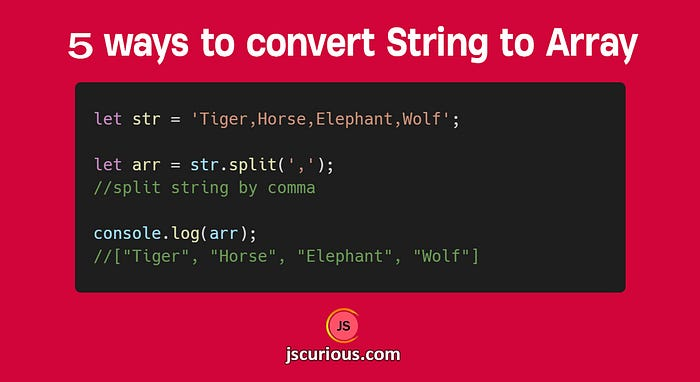# 5 Ways to Convert String to Array in JavaScriptPosted in jscurious.com

The String in JavaScript can be converted to an Array in 5 different ways. We will make use of `split`, `Array.from`, `spread`, `Object.assign` and `for` loop. Let's discuss all the methods in brief.

### 1. The split() method

This method is used to split a string based on a separator provided and returns an Array of substrings.

``````let str = 'Tiger,Horse,Elephant,Wolf';

let arr = str.split(',');
//split string by comma

console.log(arr);
//["Tiger", "Horse", "Elephant", "Wolf"]
``````

If you want to split the string by each character, then you can specify an empty string (“”) as the separator.

``````let str = 'jscurious';

let arr = str.split('');

console.log(arr);
// ["j", "s", "c", "u", "r", "i", "o", "u", "s"]
``````

The `split()` method accepts a second optional argument which sets the limit on the splits. This limit value decides how many elements will be included in the returned array.

``````let str = 'Cricket | Hockey | Football | Tennis';

let arr = str.split(' | ', 2);

console.log(arr);
// ['Cricket', 'Hockey']
``````

### 2. The Array.from() method

The `Array.from()` method returns an Array from any iterable object. We can pass a string value in `from()` method to get a character array.

``````let str = 'jscurious';

let arr = Array.from(str);

console.log(arr);
// ["j", "s", "c", "u", "r", "i", "o", "u", "s"]
``````

This method also accepts two optional arguments. One is a `map` function to call on each element of the array and the other one is a value to use as `this` while executing the `map` function.

``````let str = 'jscurious';

let arr = Array.from(str, (val, index) => val + index);
// adding index value to each element of array

console.log(arr);
// ["j0", "s1", "c2", "u3", "r4", "i5", "o6", "u7", "s8"]
``````

The spread operator (…) extracts and spreads each character of a String. We can just wrap all those characters inside an array literal to create a new array from the string.

``````let str = 'jscurious';

let arr = [...str];

console.log(arr);
// ["j", "s", "c", "u", "r", "i", "o", "u", "s"]
``````

### 4. The Object.assign() method

This method is used to copy the values and properties from one or more source objects to a target object. We can provide a string as the source and an empty array as the target to create an array from the string.

``````let str = 'jscurious';

let arr = Object.assign([], str);

console.log(arr);
// ["j", "s", "c", "u", "r", "i", "o", "u", "s"]
``````

### 5. The For loop

We can loop through each character of a string using for loop and push that character to an empty array to create an array from the string.

``````let str = 'jscurious';

let arr = [];

for(let i of str) {
arr.push(i);
}

console.log(arr);
// ["j", "s", "c", "u", "r", "i", "o", "u", "s"]
``````

That's it for this topic. Thank you for reading.